# Analyzing rtweet Data with kerasformula

The kerasformula package offers a high-level interface for the R interface to Keras. It’s main interface is the kms function, a regression-style interface to keras_model_sequential that uses formulas and sparse matrices. We use kerasformula to predict how popular tweets will be based on how often the tweet was retweeted and favorited.

01-24-2018

## Overview

The kerasformula package offers a high-level interface for the R interface to Keras. It’s main interface is the `kms` function, a regression-style interface to `keras_model_sequential` that uses formulas and sparse matrices.

The kerasformula package is available on CRAN, and can be installed with:

``````# install the kerasformula package
install.packages("kerasformula")
# or devtools::install_github("rdrr1990/kerasformula")

library(kerasformula)

# install the core keras library (if you haven't already done so)
# see ?install_keras() for options e.g. install_keras(tensorflow = "gpu")
install_keras()``````

## The kms() function

Many classic machine learning tutorials assume that data come in a relatively homogenous form (e.g., pixels for digit recognition or word counts or ranks) which can make coding somewhat cumbersome when data is contained in a heterogenous data frame. `kms()` takes advantage of the flexibility of R formulas to smooth this process.

`kms` builds dense neural nets and, after fitting them, returns a single object with predictions, measures of fit, and details about the function call. `kms` accepts a number of parameters including the loss and activation functions found in `keras`. `kms` also accepts compiled `keras_model_sequential` objects allowing for even further customization. This little demo shows how `kms` can aid is model building and hyperparameter selection (e.g., batch size) starting with raw data gathered using `library(rtweet)`.

Let’s look at #rstats tweets (excluding retweets) for a six-day period ending January 24, 2018 at 10:40. This happens to give us a nice reasonable number of observations to work with in terms of runtime (and the purpose of this document is to show syntax, not build particularly predictive models).

``````rstats <- search_tweets("#rstats", n = 10000, include_rts = FALSE)
dim(rstats)``````
``   2840   42``

Suppose our goal is to predict how popular tweets will be based on how often the tweet was retweeted and favorited (which correlate strongly).

``cor(rstats\$favorite_count, rstats\$retweet_count, method="spearman")``
``     0.7051952``

Since few tweeets go viral, the data are quite skewed towards zero.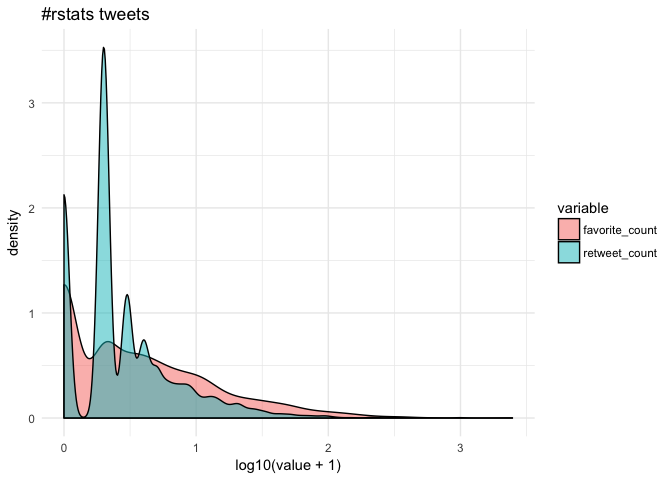## Getting the most out of formulas

Let’s suppose we are interested in putting tweets into categories based on popularity but we’re not sure how finely-grained we want to make distinctions. Some of the data, like `rstats\$mentions_screen_name` comes in a list of varying lengths, so let’s write a helper function to count non-NA entries.

``````n <- function(x) {
unlist(lapply(x, function(y){length(y) - is.na(y)}))
}``````

Let’s start with a dense neural net, the default of `kms`. We can use base R functions to help clean the data–in this case, `cut` to discretize the outcome, `grepl` to look for key words, and `weekdays` and `format` to capture different aspects of the time the tweet was posted.

``````breaks <- c(-1, 0, 1, 10, 100, 1000, 10000)
popularity <- kms(cut(retweet_count + favorite_count, breaks) ~ screen_name +
source + n(hashtags) + n(mentions_screen_name) +
n(urls_url) + nchar(text) +
grepl('photo', media_type) +
weekdays(created_at) +
format(created_at, '%H'), rstats)
plot(popularity\$history)
+ ggtitle(paste("#rstat popularity:",
paste0(round(100*popularity\$evaluations\$acc, 1), "%"),
"out-of-sample accuracy"))
+ theme_minimal()

popularity\$confusion``````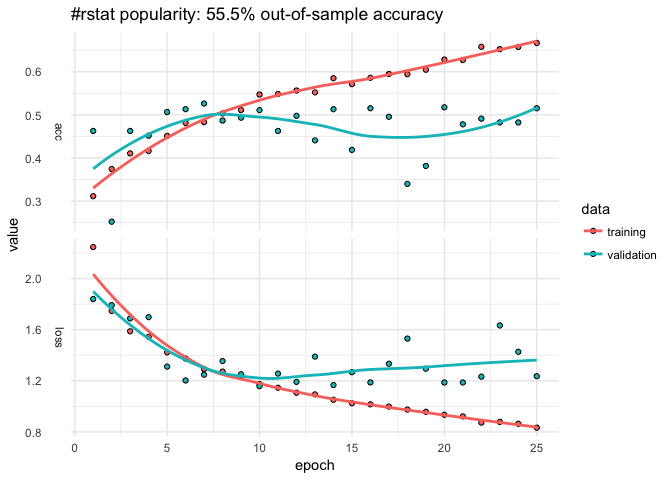``````popularity\$confusion

(-1,0] (0,1] (1,10] (10,100] (100,1e+03] (1e+03,1e+04]
(-1,0]            37    12     28        2           0             0
(0,1]             14    19     72        1           0             0
(1,10]             6    11    187       30           0             0
(10,100]           1     3     54       68           0             0
(100,1e+03]        0     0      4       10           0             0
(1e+03,1e+04]      0     0      0        1           0             0``````

The model only classifies about 55% of the out-of-sample data correctly and that predictive accuracy doesn’t improve after the first ten epochs. The confusion matrix suggests that model does best with tweets that are retweeted a handful of times but overpredicts the 1-10 level. The `history` plot also suggests that out-of-sample accuracy is not very stable. We can easily change the breakpoints and number of epochs.

``````breaks <- c(-1, 0, 1, 25, 50, 75, 100, 500, 1000, 10000)
popularity <- kms(cut(retweet_count + favorite_count, breaks) ~
n(hashtags) + n(mentions_screen_name) + n(urls_url) +
nchar(text) +
screen_name + source +
grepl('photo', media_type) +
weekdays(created_at) +
format(created_at, '%H'), rstats, Nepochs = 10)

plot(popularity\$history)
+ ggtitle(paste("#rstat popularity (new breakpoints):",
paste0(round(100*popularity\$evaluations\$acc, 1), "%"),
"out-of-sample accuracy"))
+ theme_minimal()``````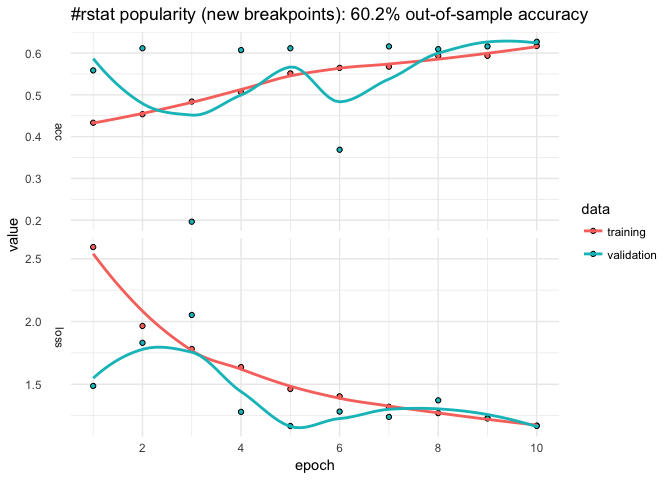That helped some (about 5% additional predictive accuracy). Suppose we want to add a little more data. Let’s first store the input formula.

``````pop_input <- "cut(retweet_count + favorite_count, breaks) ~
n(hashtags) + n(mentions_screen_name) + n(urls_url) +
nchar(text) +
screen_name + source +
grepl('photo', media_type) +
weekdays(created_at) +
format(created_at, '%H')"``````

Here we use `paste0` to add to the formula by looping over user IDs adding something like:

``grepl("12233344455556", mentions_user_id)``
``````mentions <- unlist(rstats\$mentions_user_id)
mentions <- unique(mentions[which(table(mentions) > 5)]) # remove infrequent
mentions <- mentions[!is.na(mentions)] # drop NA

for(i in mentions)
pop_input <- paste0(pop_input, " + ", "grepl(", i, ", mentions_user_id)")

popularity <- kms(pop_input, rstats)``````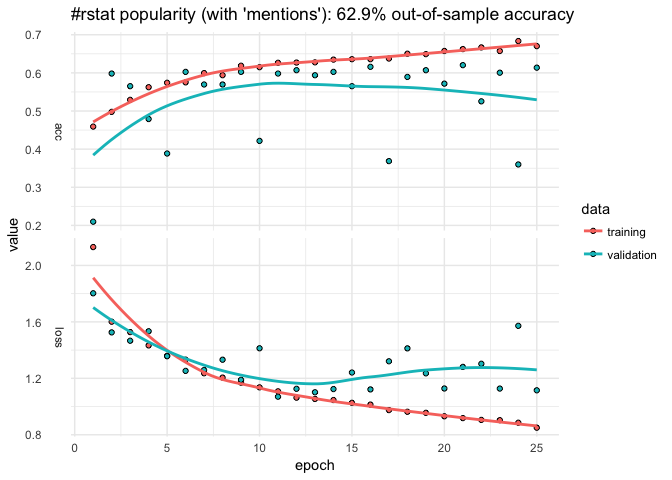That helped a touch but the predictive accuracy is still fairly unstable across epochs…

## Customizing layers with kms()

We could add more data, perhaps add individual words from the text or some other summary stat (`mean(text %in% LETTERS)` to see if all caps explains popularity). But let’s alter the neural net.

The `input.formula` is used to create a sparse model matrix. For example, `rstats\$source` (Twitter or Twitter-client application type) and `rstats\$screen_name` are character vectors that will be dummied out. How many columns does it have?

``popularity\$P``
``     1277``

Say we wanted to reshape the layers to transition more gradually from the input shape to the output.

``````popularity <- kms(pop_input, rstats,
layers = list(
units = c(1024, 512, 256, 128, NA),
activation = c("relu", "relu", "relu", "relu", "softmax"),
dropout = c(0.5, 0.45, 0.4, 0.35, NA)
))``````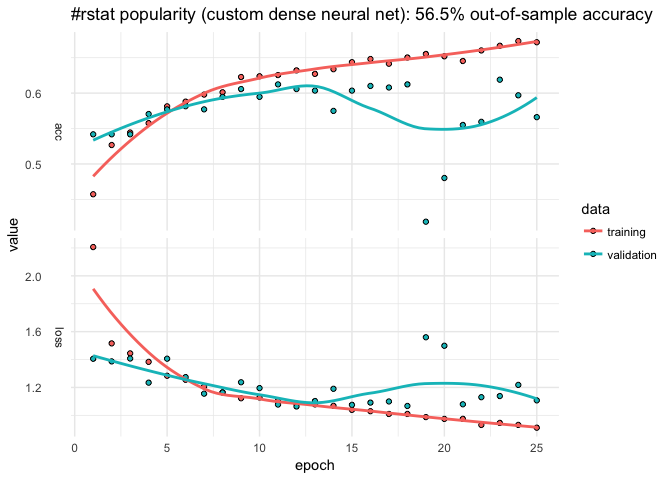`kms` builds a `keras_sequential_model()`, which is a stack of linear layers. The input shape is determined by the dimensionality of the model matrix (`popularity\$P`) but after that users are free to determine the number of layers and so on. The `kms` argument `layers` expects a list, the first entry of which is a vector `units` with which to call `keras::layer_dense()`. The first element the number of `units` in the first layer, the second element for the second layer, and so on (`NA` as the final element connotes to auto-detect the final number of units based on the observed number of outcomes). `activation` is also passed to `layer_dense()` and may take values such as `softmax`, `relu`, `elu`, and `linear`. (`kms` also has a separate parameter to control the optimizer; by default `kms(... optimizer = 'rms_prop')`.) The `dropout` that follows each dense layer rate prevents overfitting (but of course isn’t applicable to the final layer).

## Choosing a Batch Size

By default, `kms` uses batches of 32. Suppose we were happy with our model but didn’t have any particular intuition about what the size should be.

``````Nbatch <- c(16, 32, 64)
Nruns <- 4
accuracy <- matrix(nrow = Nruns, ncol = length(Nbatch))
colnames(accuracy) <- paste0("Nbatch_", Nbatch)

est <- list()
for(i in 1:Nruns){
for(j in 1:length(Nbatch)){
est[[i]] <- kms(pop_input, rstats, Nepochs = 2, batch_size = Nbatch[j])
accuracy[i,j] <- est[[i]][["evaluations"]][["acc"]]
}
}

colMeans(accuracy)``````
``````    Nbatch_16 Nbatch_32 Nbatch_64
0.5088407 0.3820850 0.5556952 ``````

For the sake of curtailing runtime, the number of epochs was set arbitrarily short but, from those results, 64 is the best batch size.

## Making predictions for new data

Thus far, we have been using the default settings for `kms` which first splits data into 80% training and 20% testing. Of the 80% training, a certain portion is set aside for validation and that’s what produces the epoch-by-epoch graphs of loss and accuracy. The 20% is only used at the end to assess predictive accuracy. But suppose you wanted to make predictions on a new data set…

``````popularity <- kms(pop_input, rstats[1:1000,])
predictions <- predict(popularity, rstats[1001:2000,])
predictions\$accuracy``````
``     0.579``
``# predictions\$confusion``

Because the formula creates a dummy variable for each screen name and mention, any given set of tweets is all but guaranteed to have different columns. `predict.kms_fit` is an `S3 method` that takes the new data and constructs a (sparse) model matrix that preserves the original structure of the training matrix. `predict` then returns the predictions along with a confusion matrix and accuracy score.

If your newdata has the same observed levels of y and columns of x_train (the model matrix), you can also use `keras::predict_classes` on `object\$model`.

## Using a compiled Keras model

This section shows how to input a model compiled in the fashion typical to `library(keras)`, which is useful for more advanced models. Here is an example for `lstm` analogous to the imbd with Keras example.

``````k <- keras_model_sequential()
k %>%
layer_embedding(input_dim = popularity\$P, output_dim = popularity\$P) %>%
layer_lstm(units = 512, dropout = 0.4, recurrent_dropout = 0.2) %>%
layer_dense(units = 256, activation = "relu") %>%
layer_dropout(0.3) %>%
layer_dense(units = 8, # number of levels observed on y (outcome)
activation = 'sigmoid')

k %>% compile(
loss = 'categorical_crossentropy',
optimizer = 'rmsprop',
metrics = c('accuracy')
)

popularity_lstm <- kms(pop_input, rstats, k)``````

Drop me a line via the project’s Github repo. Special thanks to @dfalbel and @jjallaire for helpful suggestions!!

### Corrections

If you see mistakes or want to suggest changes, please create an issue on the source repository.

### Citation

`Mohanty (2018, Jan. 24). Posit AI Blog: Analyzing rtweet Data with kerasformula. Retrieved from https://blogs.rstudio.com/tensorflow/posts/2018-01-24-analyzing-rtweet-data-with-kerasformula/`
```@misc{mohanty2018analyzing,